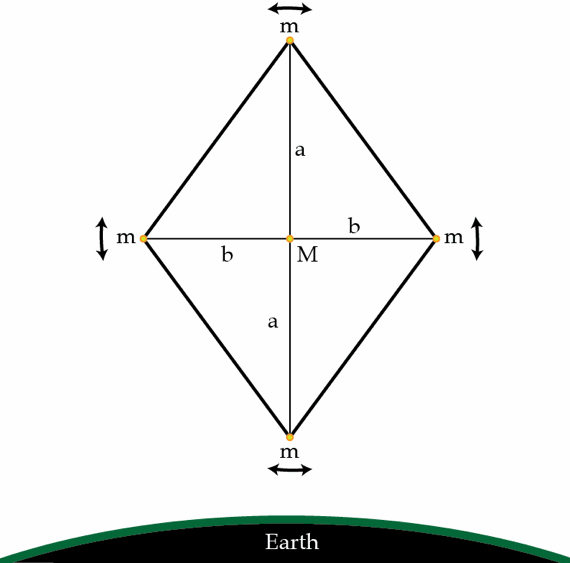# Sleeping Satellite

Consider a small geostationary satellite consisting of four point masses $m$ connected by light rigid rods of length $a$ and $b$ to a central mass $M$ ($M\gg m$) as shown in the figure. In addition, four light rods of length $\sqrt{a^{2}+b^{2}}$ ensure that the satellite behaves as rigid body. It turns out that if $\frac{a}{b}>1$, the satellite can oscillate about its center of mass in the plane of the orbit. In other words, the configuration showed in the figure is stable. For what ratio $\eta=\frac{a}{b}$ the period of small oscillations of the satellite equals its orbital period? Assume that the dimensions of the satellite are much smaller than the radius its orbit.×